## Monte Carlo Simulation

On a nice day 2 years ago, when I was on financial field. My boss sent our team an email. In this email, he would like to us propose some machine learning techniques to predict stock price.

So, after accepting the assignment from my manager, our team begin to research and apply some approaches for prediction. When we talk about Machine Learning, we often think of supervised and unsupervised learning. But one of the algorithms we applied is one that we usually forgotten  however equally highly effective algorithm: Monte Carlo Simulation.

## What is Monte Carlo simulation

Monte Carlo method is a technique that uses random numbers and probability to solve complex problems. The Monte Carlo simulation, or probability simulation, is a technique used to understand the impact of risk and uncertainty in financial sectors, project management, costs, and other forecasting machine learning models.

Now let’s jump into python implementation to see how it applies,

## Python Implementation

In this task we used data of DXG stock dataset from 2017/01/01 to 2018/08/24 and we would like to know what is stock price after 10 days, 1 months and 3 months, respectively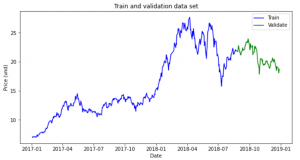We will simulate the return of stock and next price will be calculated by

P(t) = P(0) * (1+return_simulate(t))

Calculate mean and standard deviation of stock returns

```miu = np.mean(stock_returns, axis=0)
dev = np.std(stock_returns)```

Simulation process

```simulation_df = pd.DataFrame()
last_price = init_price
for x in range(mc_rep):
count = 0
daily_vol = dev
price_series = []
price = last_price * (1 + np.random.normal(miu, daily_vol))
price_series.append(price)
for y in range(train_days):
if count == train_days-1:
break
price = price_series[count] * (1 + np.random.normal(miu, daily_vol))
price_series.append(price)
count += 1
simulation_df[x] = price_series```

Visualization Monte Carlo Simulation

```fig = plt.figure()
fig.suptitle('Monte Carlo Simulation')
plt.plot(simulation_df)
plt.axhline(y = last_price, color = 'r', linestyle = '-')
plt.xlabel('Day')
plt.ylabel('Price')
plt.show()```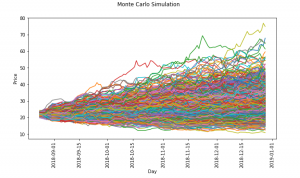Now, let’s check with actual stock price after 10 days, 1 month and 3 months

```plt.hist(simulation_df.iloc[9,:],bins=15,label ='histogram')
plt.axvline(x = test_simulate.iloc, color = 'r', linestyle = '-',label ='Price at 10th')
plt.legend()
plt.title('Histogram simulation and last price of 10th day')
plt.show()```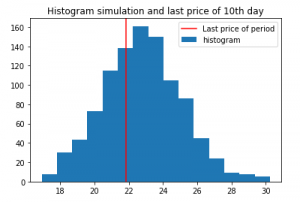We can see the most frequent occurrence price is pretty close with the actual price after 10th

If the forecast period is longer, the results is not good gradually

Simulation for next 1 month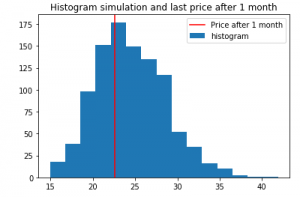After 3 months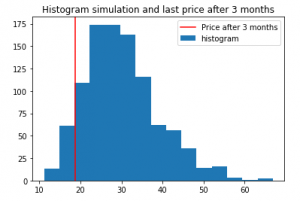## Conclusion

Monte Carlo simulation is used a lot in finance, although it has some weaknesses, but hopefully through this article, you will have a new look on the simulation application for forecasting.

## Reference

 Pratik Shukla, Roberto Iriondo, “Monte Carlo Simulation An In-depth Tutorial with Python”, medium, https://medium.com/towards-artificial-intelligence/monte-carlo-simulation-an-in-depth-tutorial-with-python-bcf6eb7856c8

Please also check Gaussian Samples and N-gram language models,
Bayesian Statistics  for statistics knowledges.